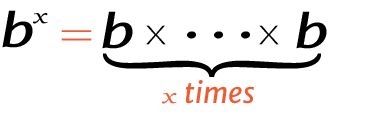# -2 raise to the power of -2.5

Place the base and exponent in the power calculator to get the right answer for value of -2.5^-2, -2.5-2 (-2.5 power -2), or -2 raised to the power of -2.5.

## How to calculate -2.5 raise to the power of -2?

This is how you can solve (-2.5) -2 manually.

Solution:

Step 1: Simplify the data.

Base
ExponentStep 2: Take the reciprocal of the value to make the exponent positive.

1-2.5(-2)

Note: Exponential calculator automatically detects the positive exponents and negative exponents.

Step 3: Multiply the -2.5 to itself -2 times.

Using this exponents calculator could be much easier to figure out an exponential expression.